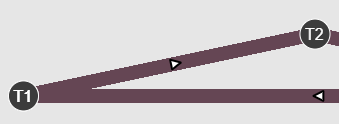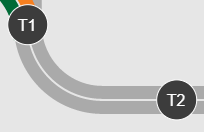# Low Resolution Lines

Hi all,

I have an issue with MultiLines plotting at low resolution (think Microsoft Paint). It isn’t a constant issue, but is bothering nonetheless. The first two figures below are both created in the exact same way (they use the same function), the only difference is in the top figure (the fuzzy one) only the endpoints are provided (T1 and T2), whereas the bottom figure contains the interpolated points (100 points between T1 and T2). I don’t see why this difference would have an impact on the line resolution, so if anyone has some tips I’d really appreciate it.If needed I can provide more of the code, but here is the basic call that both figures use:

``````    p = figure(tools=TOOLS, plot_width=plot_width, plot_height=plot_height, x_range=x_range, y_range=y_range,
toolbar_location=None, sizing_mode='scale_width', aspect_scale=1, css_classes=['schematic'],
name='schematic')

# schematic
g1 = p.multi_line('x', 'y', source=source, line_color=mapper, line_width=line_width, alpha=line_alpha)

selected1 = MultiLine(line_alpha=line_alpha, line_color=mapper, line_width=line_width)
nonselected1 = MultiLine(line_alpha=line_alpha, line_color=mapper, line_width=line_width)

g1.selection_glyph = selected1
g1.nonselection_glyph = nonselected1

p.background_fill_color = None
p.border_fill_color = None
p.outline_line_color = None

p.axis.visible = False
p.xgrid.grid_line_color = None
p.ygrid.grid_line_color = None
``````

What browser is this happening in? Can you check in others? Offhand this looks like the pixel ratio for a HiDPI canvas is not being set (maybe).

Hi Bryan,

I’m running Chrome. I tried it in IE11 and Edge just now and it looks the same.

Hrmm, possibly a Windows specific issue, then. If you have the ability to try to reproduce this:

• on non-windows devices
• on different windows devices

That would be a huge help to narrow things down.

I don’t have access to a Mac at work (just Windows and headless Linux), but I tested it on my Android phone (using the Chrome app) and produced the same result.

OK, can you provide a complete example so that I can run it on some other platforms?

Yeah, I’ll work up a minimal example when I get a chance.

I found the underlying issue - I had two lines stacked in the choppy figure. I’m guessing the opacity from the feathering on the edges combined and caused the edges to look lo-res. I filtered the second line out and the issue immediately went away.

1 Like